# Lectures

### LECTURE 1: INTRODUCTION I

• Overview of course material. What is concurrency and why is it important? Concurrency and the free lunch.
• Slides: [PDF]

### LECTURE 2: INTRODUCTION II

• Overview of a parallel architecture taxonomy. Data-level vs. thread-level parallelism.
• Slides: [PDF]

### LECTURE 3: OpenMP Programming I

• Introduction to implicit parallelism and OpenMP programming in C.
• Blackboard:
•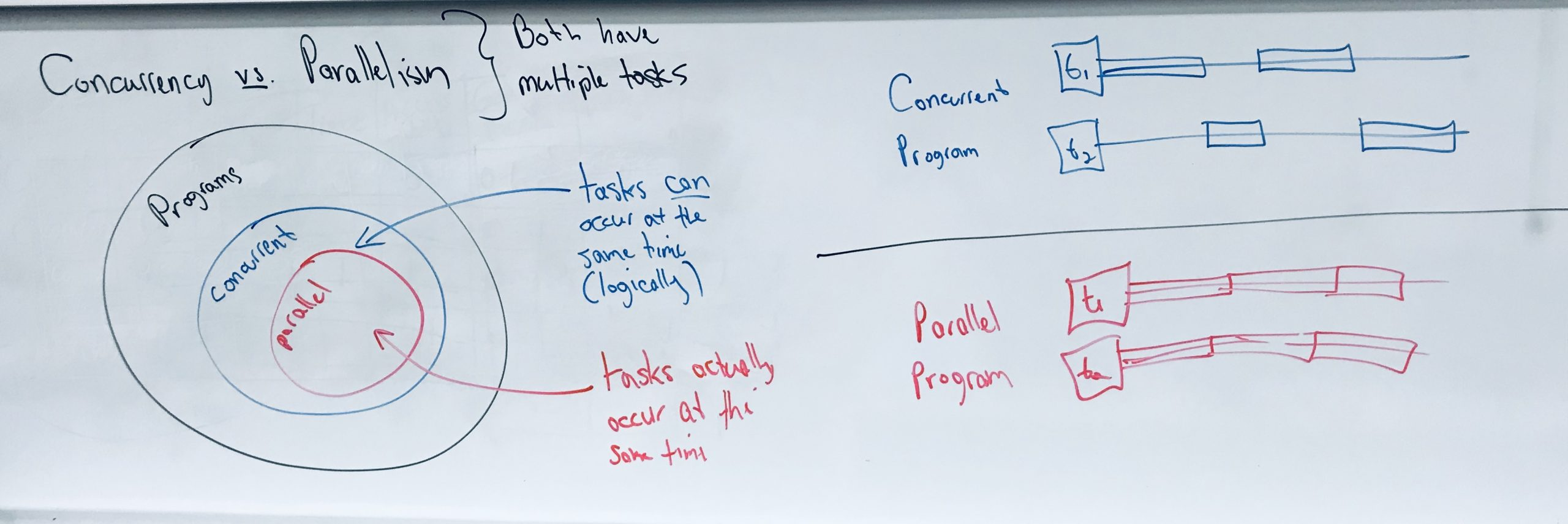•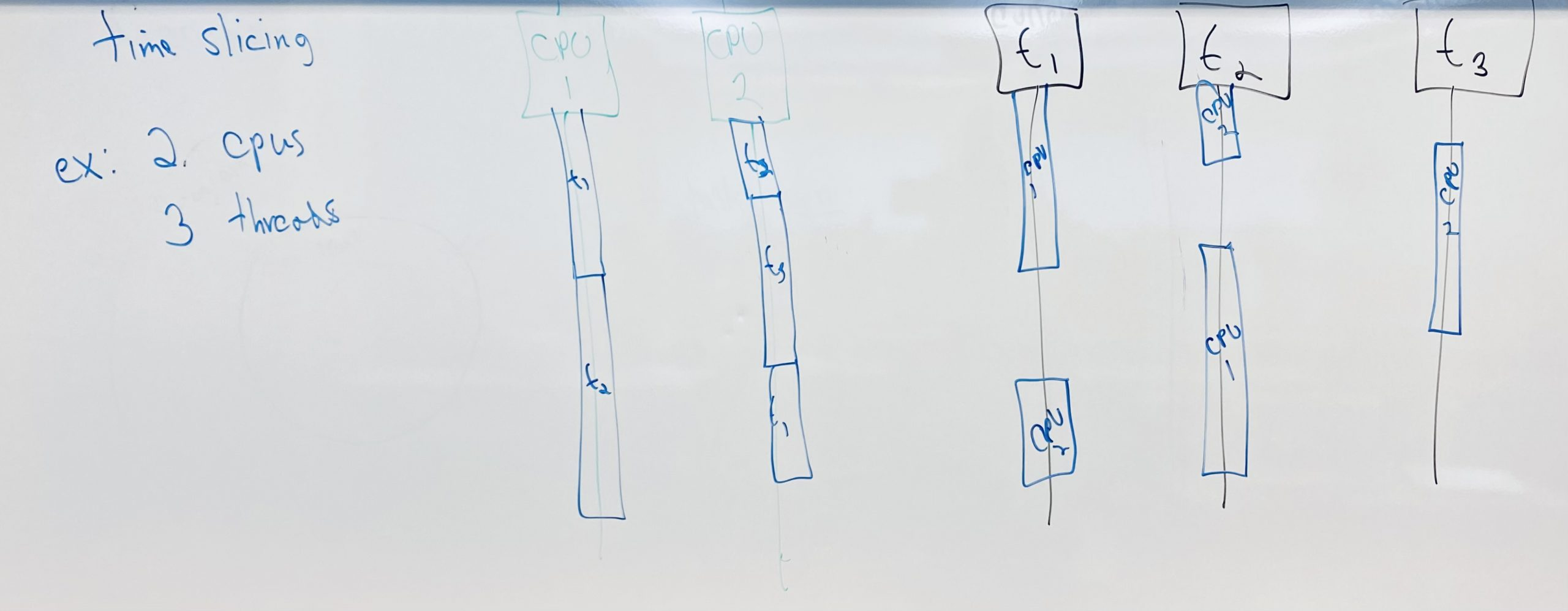•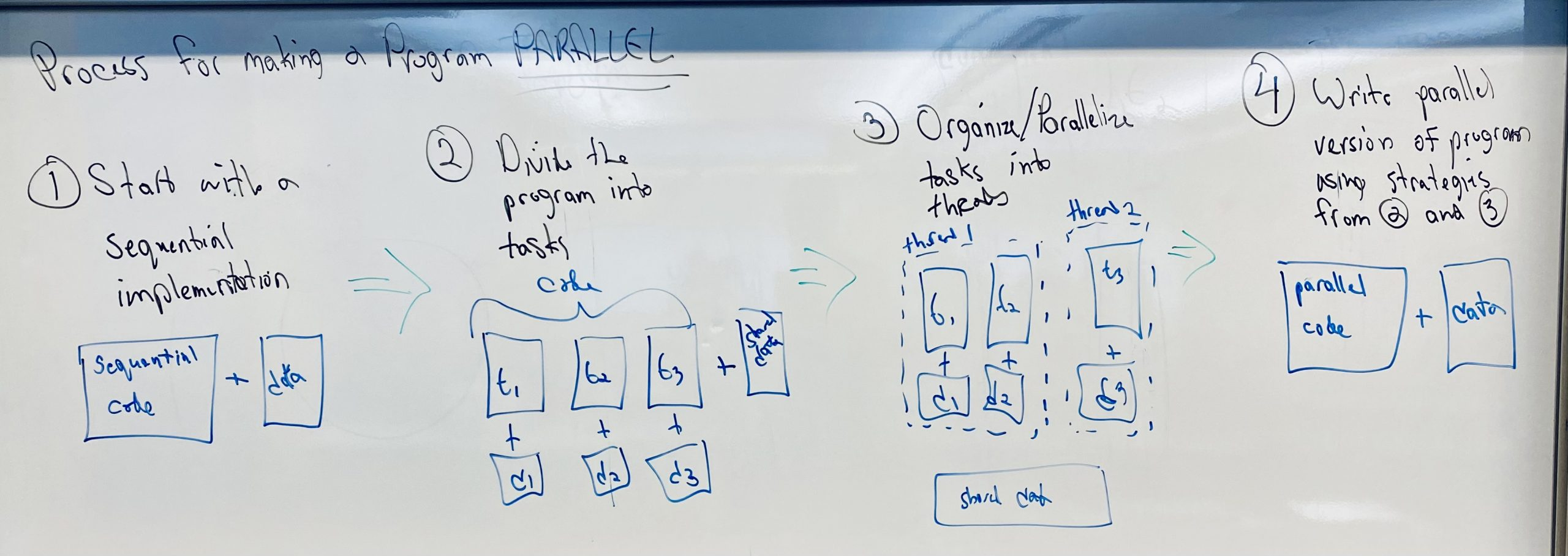• Exercises:

### LECTURE 4: OPENMP PROGRAMMING II

• Programming OpenMP with C using barriers.
• Blackboard:
•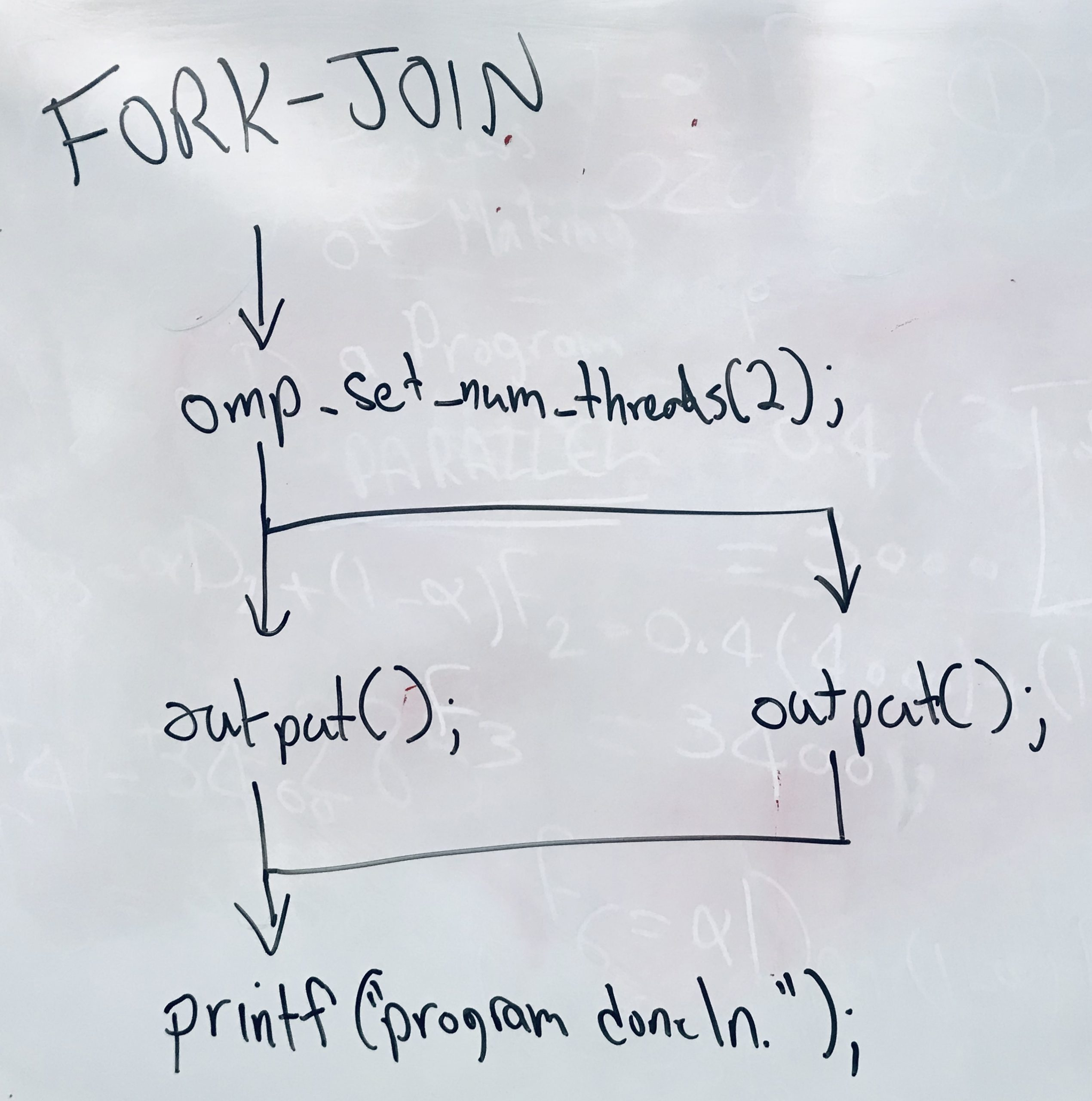•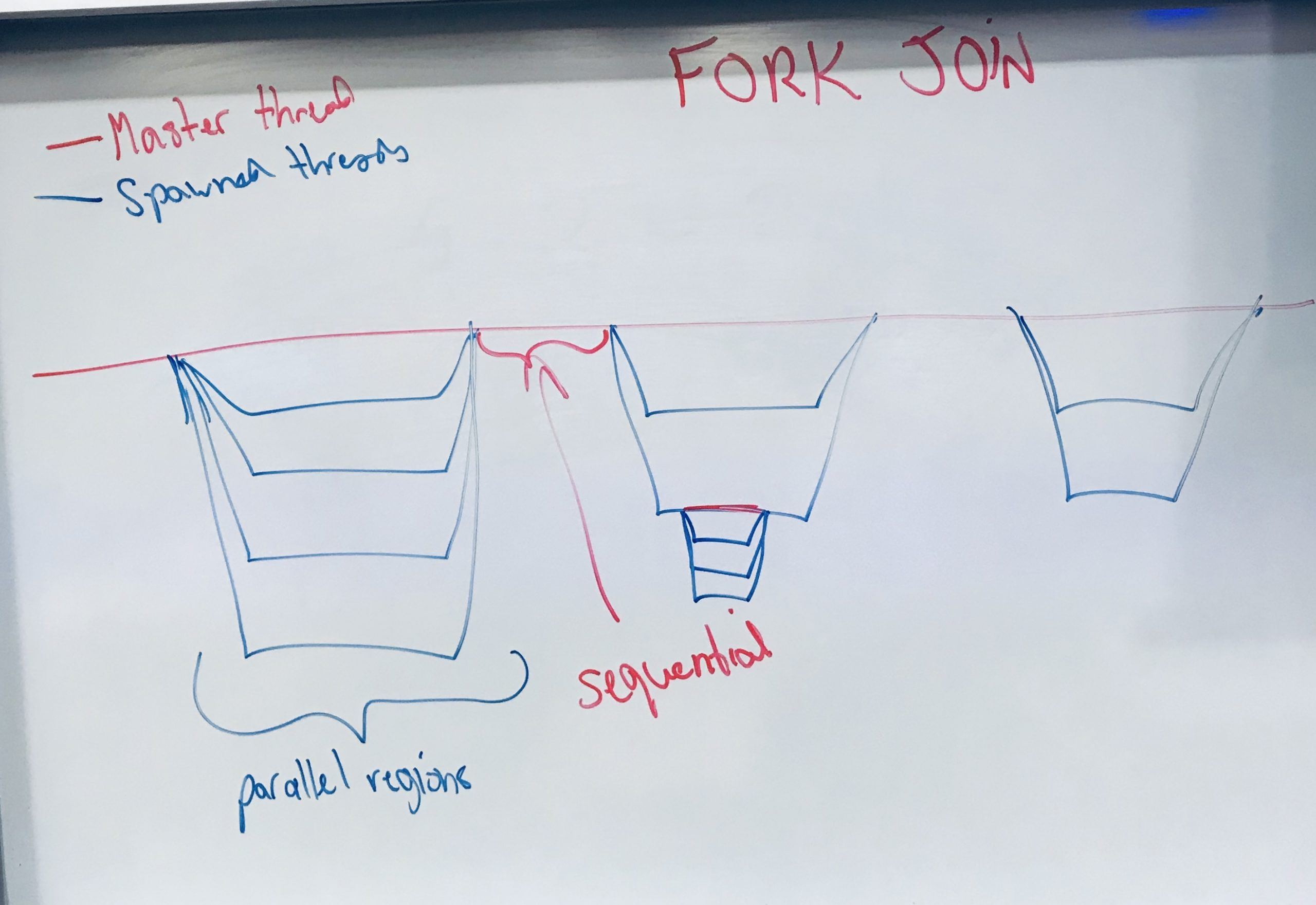• Exercises:
• barrier_ex1.c, barrier_ex2.c– an example of parallelization using a barrier.
• barrier_ex3.c – a more detailed example of parallelization using a barrier to separate two calculations involving grades.

### LECTURE 5: OPENMP PROGRAMMING III

• Programming OpenMP with C using parallel directive and critical regions.
• Exercises:
• pi_serial.c – a serial program for calculating pi as the sum of area’s of rectangles under a curve.
• pi_parallel_ex1.c – parallelization of the serial pi program using the parallel directive.
• pi_parallel_ex2.c – improving the performance of pi_parallel_v1.c. The original program suffers from “false sharing” between array elements on the same cache line. This program uses an architecture specific solution (padding) to solve the problem.

### LECTURE 6: OPENMP PROGRAMMING IV

• Programming OpenMP with C using parallel directive and atomic statements.
• Whiteboard:
•
• Exercises:
• pi_parallel_ex3.c – parallelization of the serial pi program using the parallel directive and a critical region.
• pi_parallel_ex3b.c – the danger of placing critical regions in loops is demonstrated in this program. This is not a best practice :).

### LECTURE 6: OPENMP PROGRAMMING V

• We will continue our running example to introduce parallel loops.
• Whiteboard:
•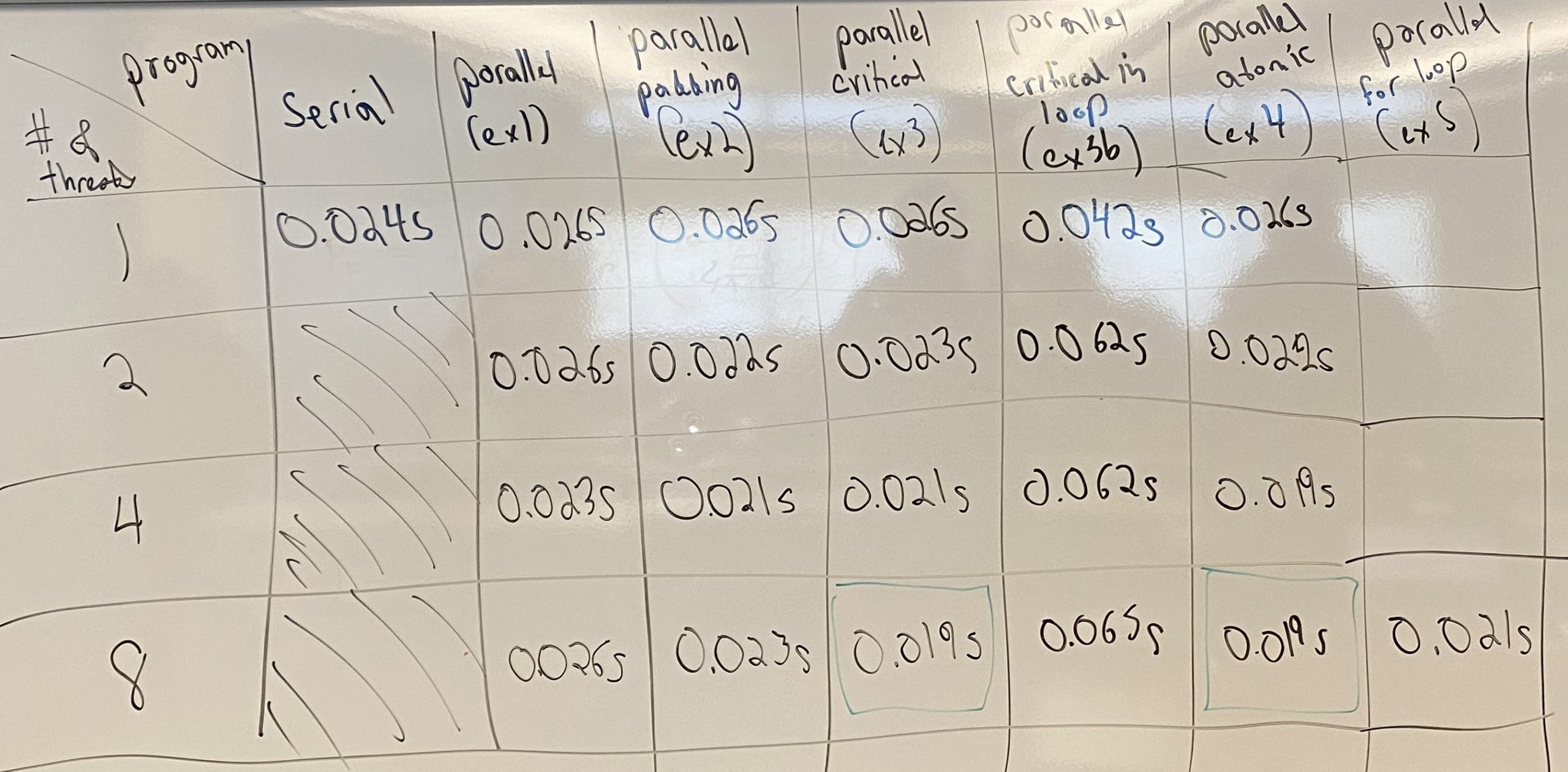• Exercises:
• pi_parallel_ex4.c – parallelization of the serial pi program using the parallel directive and an atomic statement.
• pi_parallel_ex5.c – parallelization of the serial pi program using the parallel directive and a parallel for loop (with a reduction).

### LECTURE 8: OPENMP PROGRAMMING VI

• Next we will review the expressiveness and trade-offs of atomic statements vs critical regions. First, we will review the benefits of atomic statements over critical regions. Next, we will examine the use of multiple critical regions with different names.
• Exercises:
• atomic_ex1.c – an example of the trade-offs between atomic and critical.
• critical_ex1.c – an example of two independent critical regions within the same parallel region.

### LECTURE 9: OPENMP PROGRAMMING VI

• We will now introduce loops. We will look at the process involved in parallelizing serial loops, the use of the nowait clause, nested parallel loop with the collapse clause, customizing loops with the schedule clause and finally the operators available for loop reductions (e.g., +, *, min, max).
• Whiteboard:
•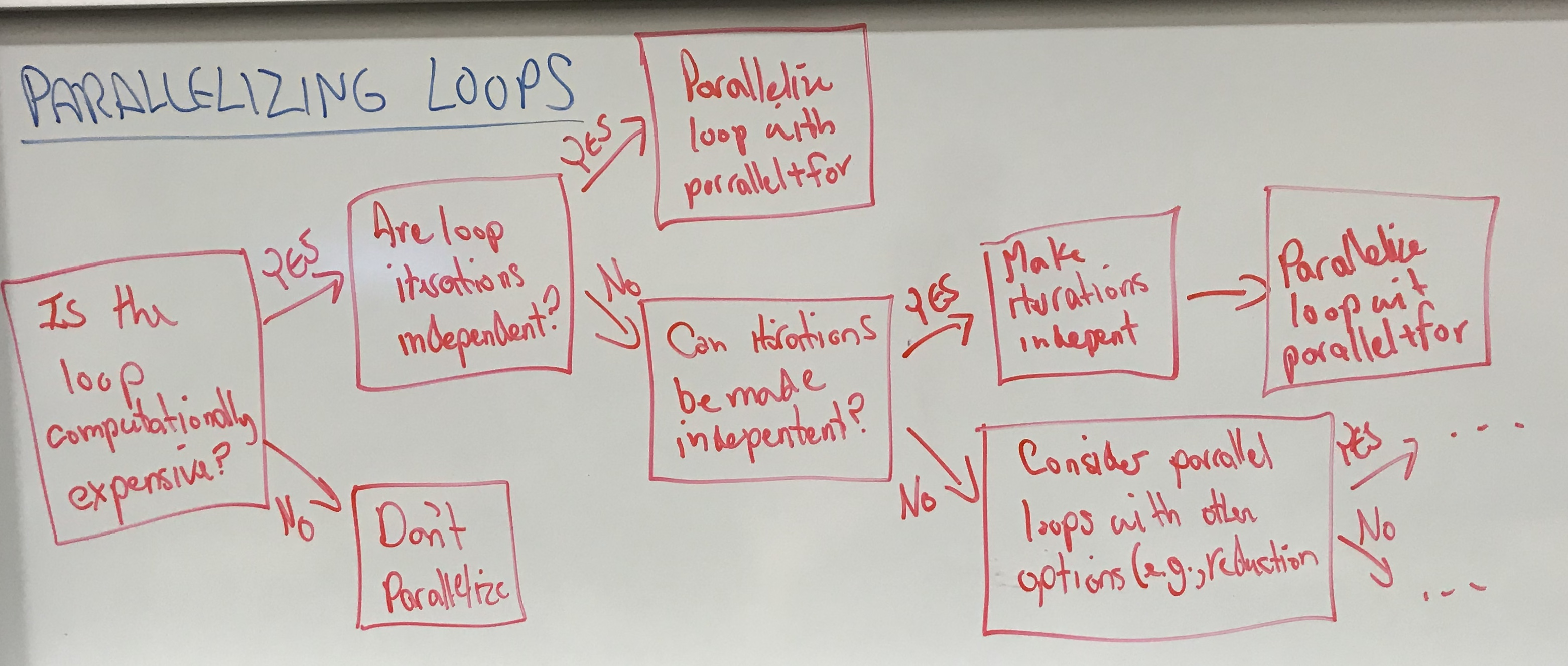• Exercises:
• loop_ex1.c – parallelizing loops by combining the parallel and for worksharing constructs.
• loop_ex2.c – an example of parallelizing a serial loop by first modifying the loop to ensure all iterations are independent.
• loop_ex3.c – an example of two parallel loops in the same parallel block that also employs the nowait clause.
• loop_ex4.c – parallelizing nested loops with the collapse clause.

### LECTURE 10: OPENMP PROGRAMMING VII [Guest Lecturer: Taylor Smith]

• Today we’ll continue to learn about loops. We will also conclude our look at OpenMP with a review of best practices.
• Blackboard:
•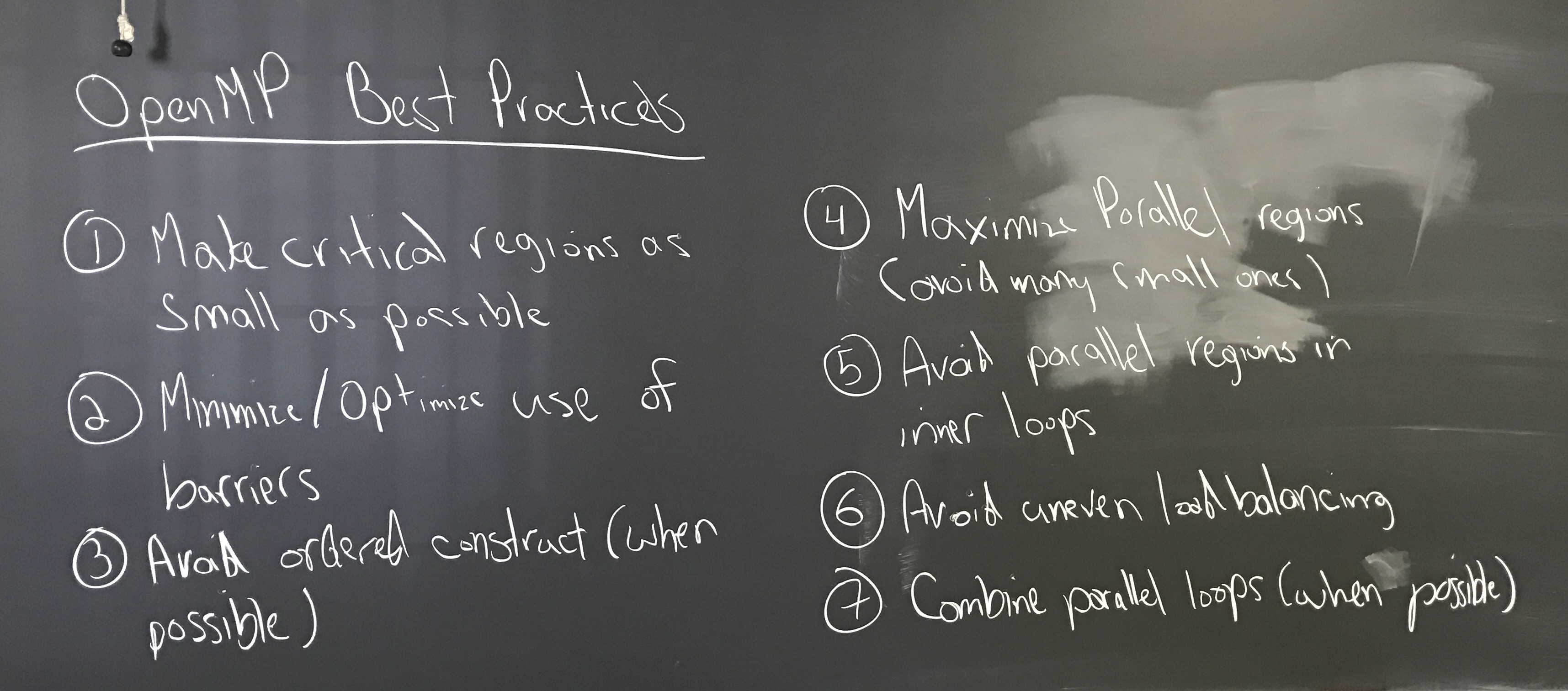• Exercises:
• loop_ex2_ordered_clause.c – an ordered clause can be used with a parallel for loop to order the output of the loop the same as if it was executed sequentially. The ordered clause does have a performance cost.
• loop_ex1_if_clause.c – an if clause can be added to a parallel construct and allows for code to be parallelized under some conditions (e.g., a high number of threads) and not parallelized under other conditions (e.g., a low number of threads).
• Notes: [PDF]

### LECTURE 11: Posix thread Programming I

• We will introduce explicit parallelism in C through discussion of threading and the pthread library. First, we will look at threads vs. processes in UNIX. Second, We will look at pthreads and explain what they are and why they are useful. We will also discuss testing threaded programs.
• Whiteboard:
•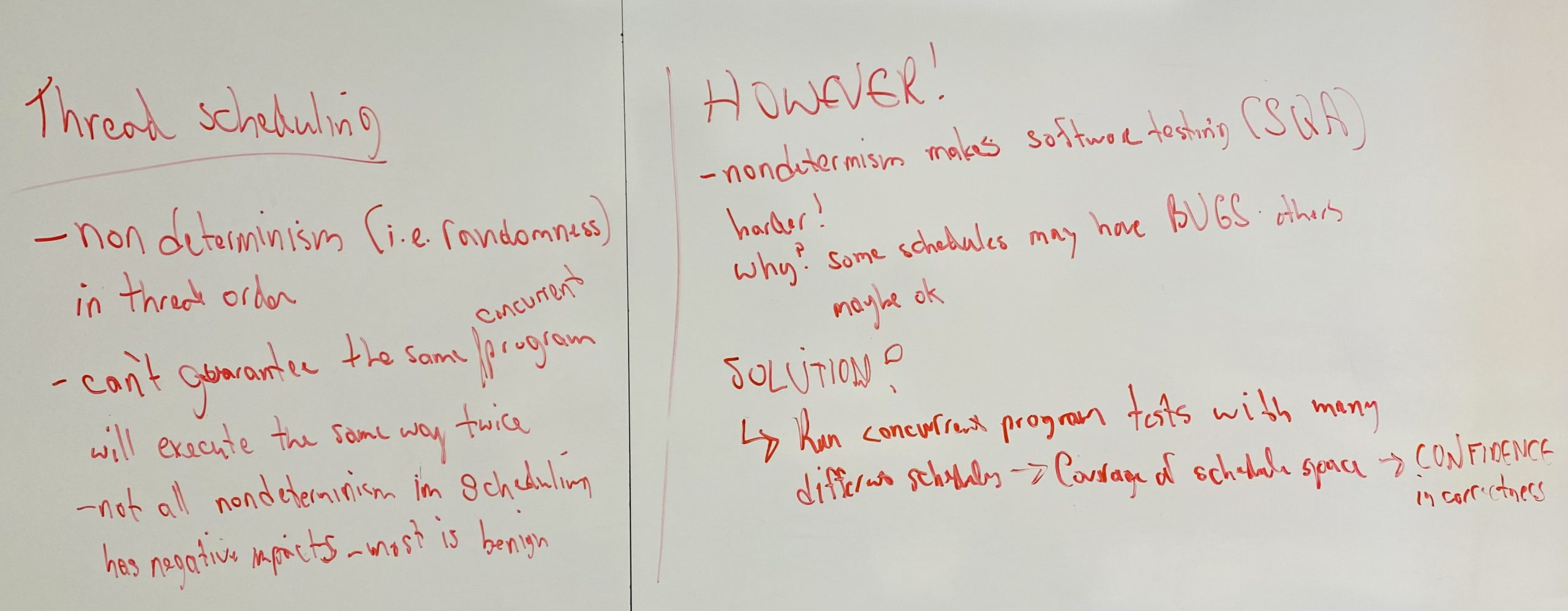•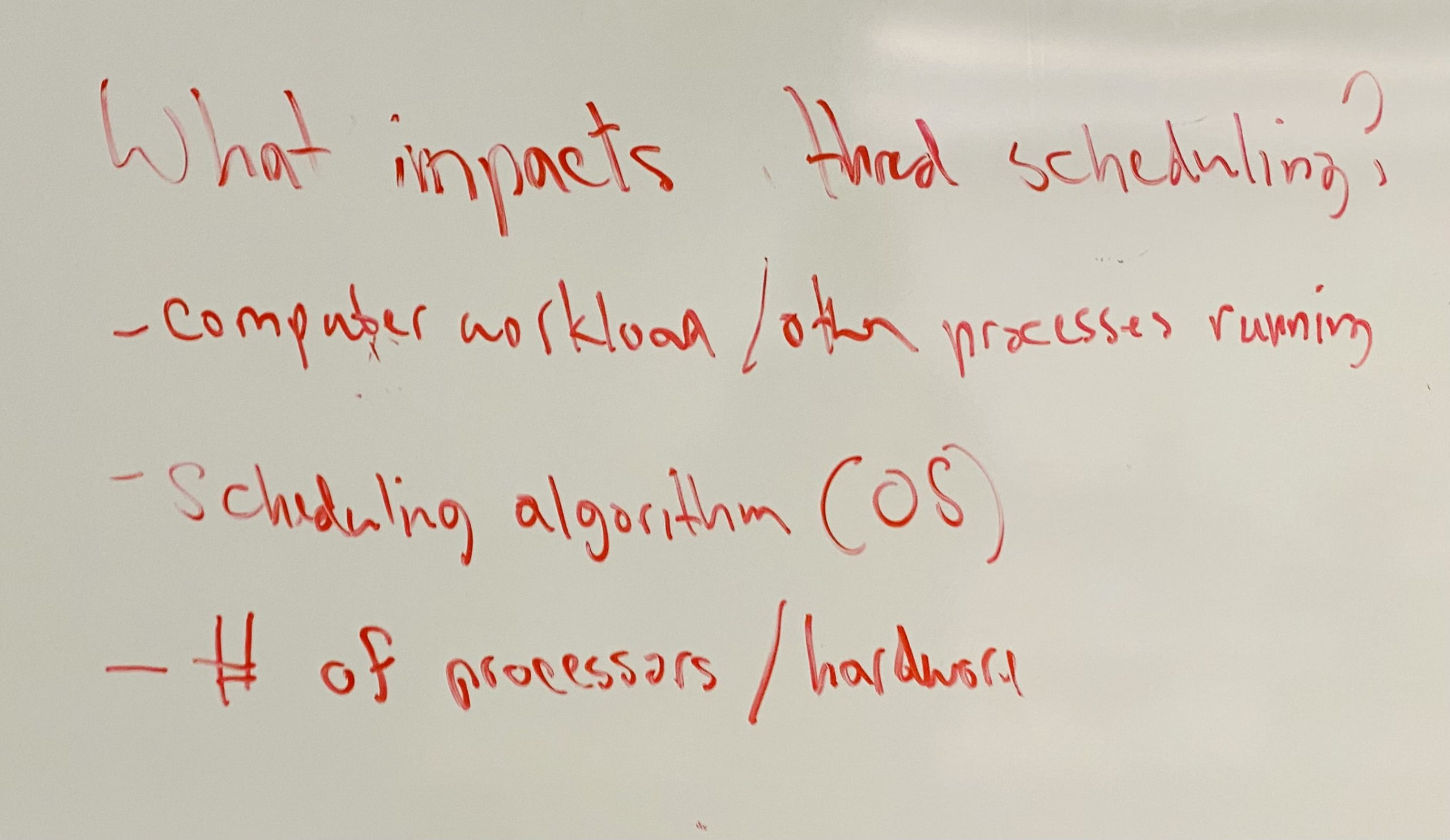•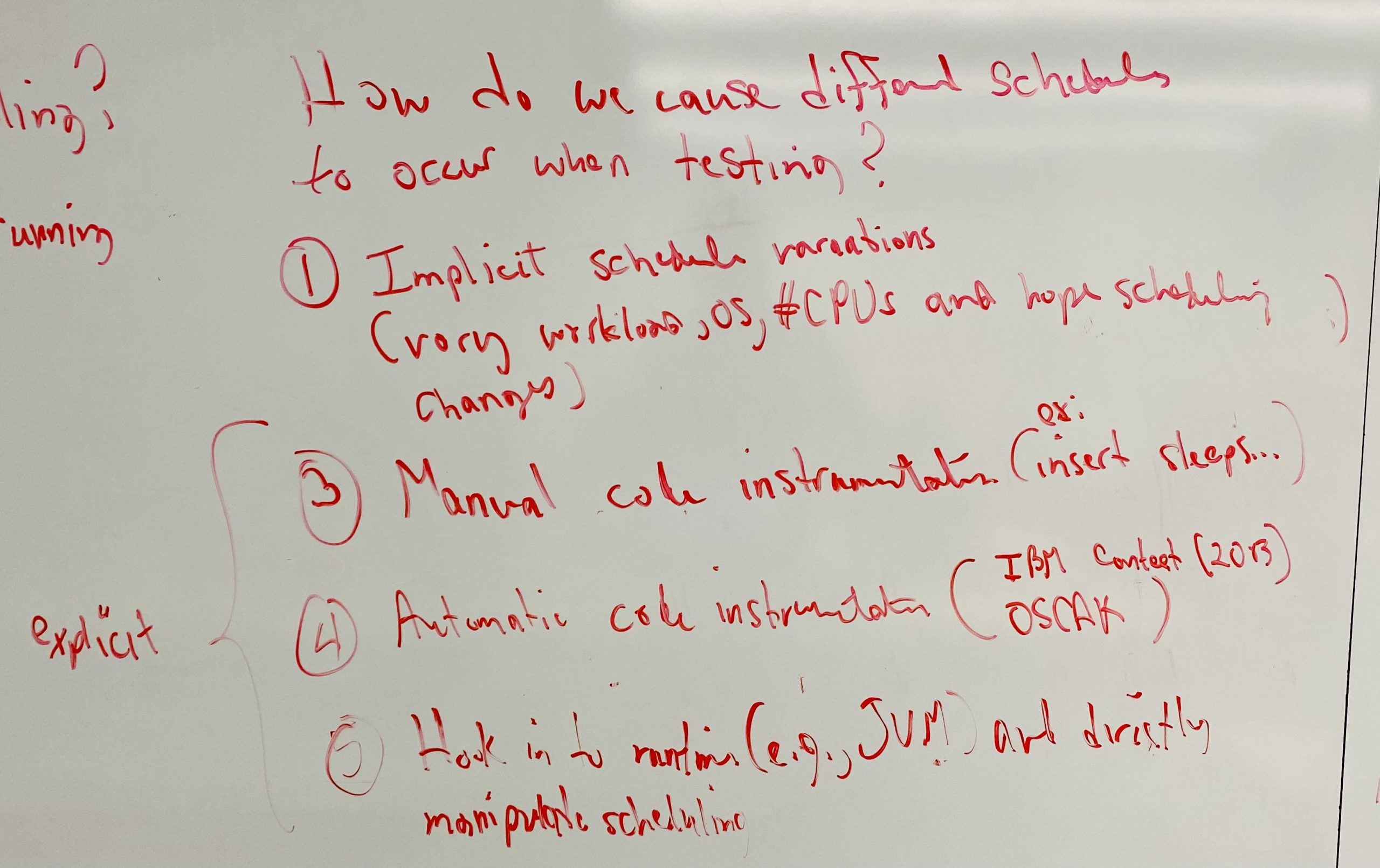• Resources:

• Exercises:
• Resources:

### LECTURE 13: POSIX THREAD PROGRAMMING III

• Accessing shared data with a pthread mutex (mutual exclusion). The difference between lock() and tryLock().
• Exercises:
• Resources:

### LECTURE 14: POSIX THREAD PROGRAMMING IV

• An in-depth look at the performance impacts of mutex, trymutex and the combined use of both.
• Blackboard:
•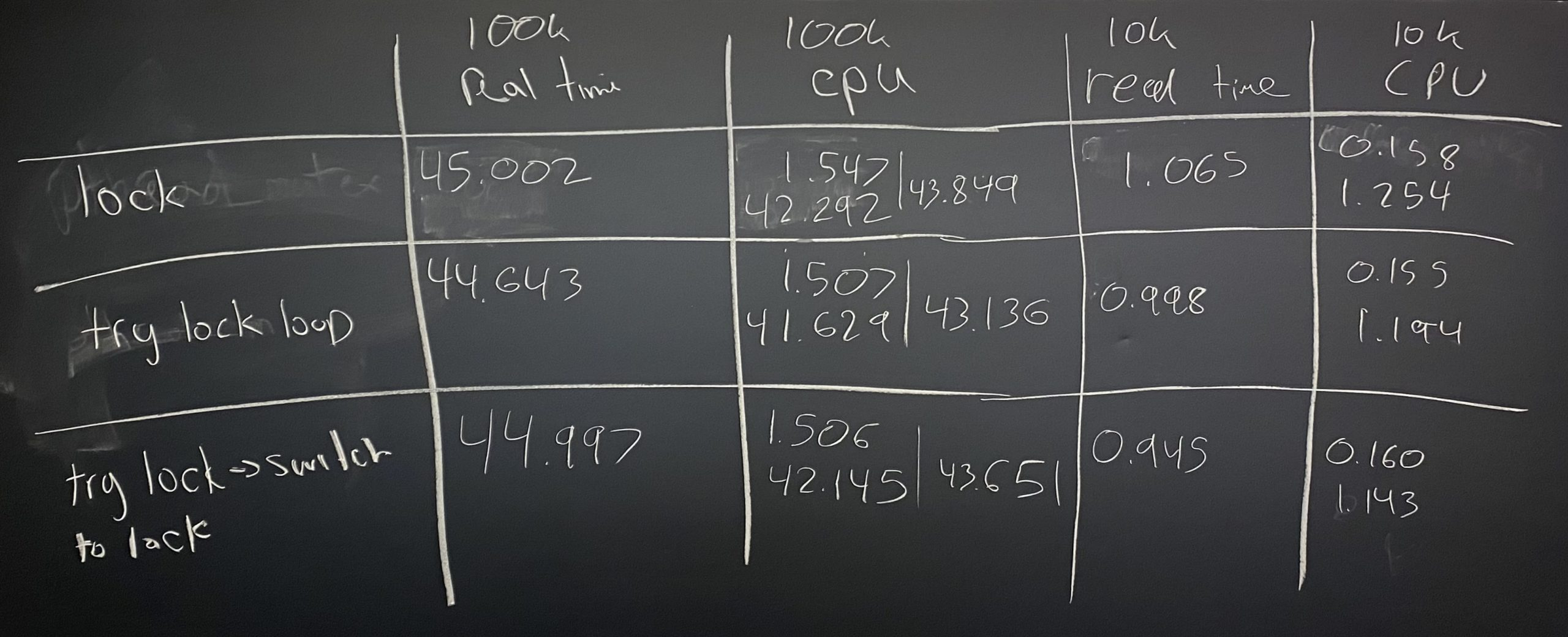• Exercises:
• pthread_trymutex3.c – an example of using a hybrid approach of a trymutex and mutex to improve performance with respect to real time and CPU time.

### LECTURE 15: POSIX THREAD PROGRAMMING V

• Pthread condition variables and condition attributes.
• Exercises:
• pthread_condition.c – an example of using a condition variable with a mutex in a stock exchange.
• Resources:

### LECTURE 16: OPENCL PROGRAMMING I

• A high-level overview of data parallelism with OpenCL.
• Blackboard:
•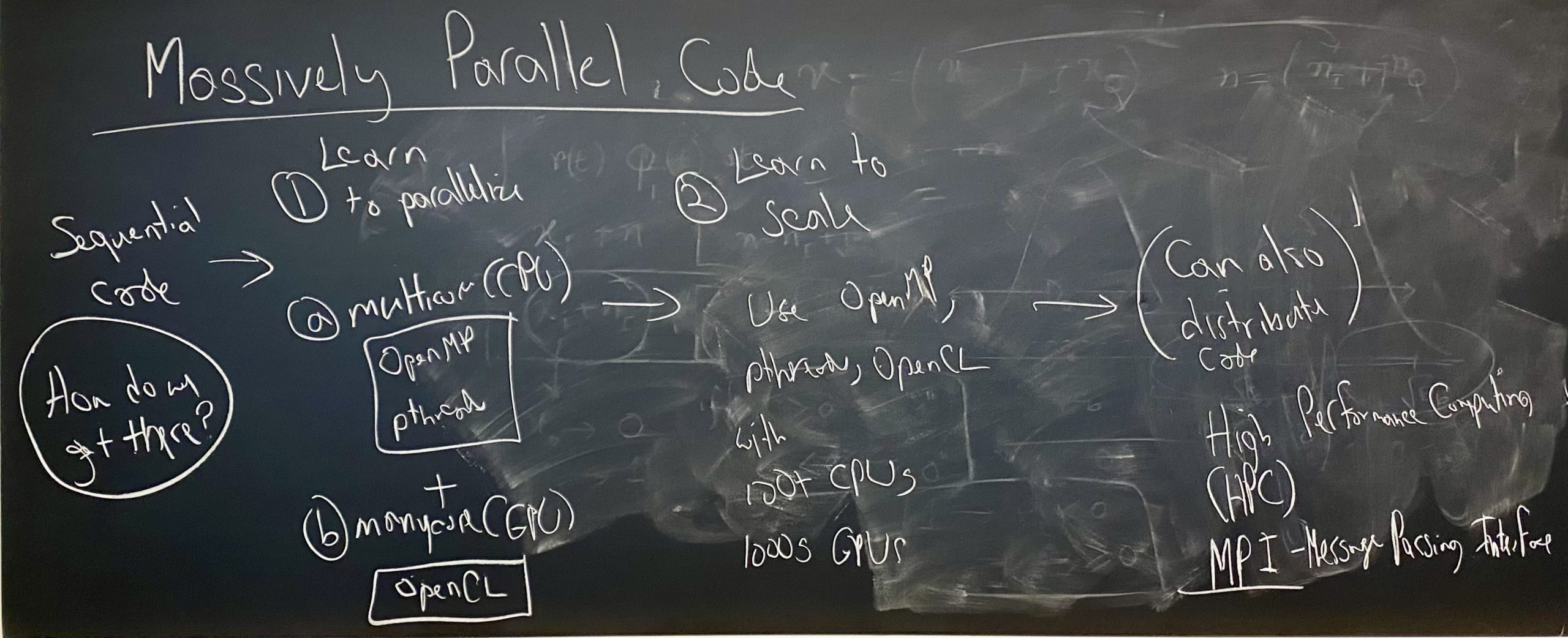• Resources:

### LECTURE 17: OPENCL PROGRAMMING II

• An introduction to memory and data in OpenCL